Comparing Quantities CBSE Class 8 worksheets ।राशियों की तुलना वर्कशीट इन हिंदी।

Ratio:
It is the quantitative relation between two quantities which reflects the relative size of both the quantities. It is simply the means to compare the quantities.

Percentage: It is another means to compare the quantities.

Finding the Increase or Decrease Percent:
To find the increase or decrease percent, multiply the percentage given by the actual quantity from which we need to increase or decrease. Then, to the actual quantity add or subtract the obtained quantity to get the final result.

Estimating Percentages:
Follow the steps given below to estimate the percentage for finding n percent of x:
(i) Round off values of x and n to numbers whose simplification would be easy.
(ii) Multiply these rounded numbers.
(iii) Divide the multiplied answer by 100.

Cost Price (CP):
The actual amount that a manufacturer spend to produce a product or to provide the service is known as the cost price

Simple Interest: If the principal remains the same for the entire loan period, then the interest paid is called simple interest. SI= P*R*T/100

Selling Price (SP):
The price at which a product is sold in the market is known as its selling price.

Profit:
The financial gain that is received on selling a product is known as its profit. In other words, it is the difference between the selling price and the cost price i.e.
Profit (P) = Selling Price (SP) – Cost Price (CP)
The profit in the percentage form can be expressed as P% = P/CP x 100.

Loss:
The financial negative revenue that is received on selling a product is known as its loss. In other words, it is the opposite of the profit.

Sales Tax (ST):
It is charge we pay to the government when we purchase items. The price of this tax is added to the selling price of an item.

How to solve comparing Qunantities.
S.P. is selling price
C.P. is cost price
The overhead expenses are a part of CP.
Profit or loss is always calculated on CP
For profit, SP>CP and for loss CP>SP
Discount is always calculated on the marked price.
VAT is not a part of selling price. Although it is calculated on the SP but it is excluded from the SP while calculating profit or loss.
Formula for compound interest gives
Amount=P(1+R/100)n
then CI=A-P
If the interest is compounded half yearly the time period becomes twice as much as the numbers of years. The rate of interest is reduced to half.
We compare two quantites by division.It is called ratio. Quantities written in same unit. Ratio has no unit
Another way to compare quantity is to express in percentages.
Profit= Profit/CPX100, Loss= Loss/CpX100

Comparing Quantities worksheets । राशियों                                          की तुलना वर्कशीट इन हिंदी।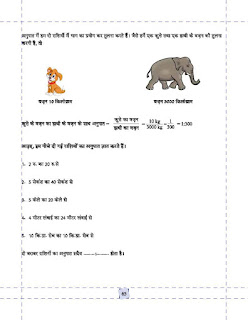Comparing Quantities worksheets in HindiComparing Quantities worksheets in HindiComparing Quantities worksheets in Hindi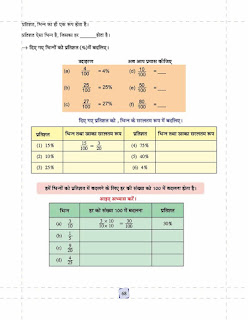Percentage worksheet in Hindi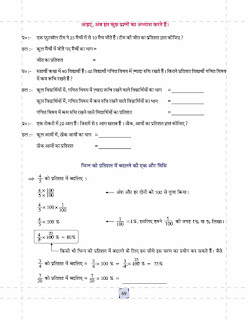Percentage worksheet in Hindi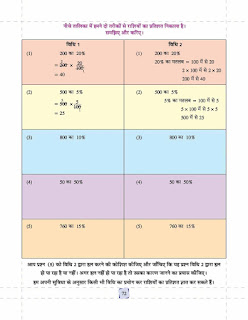Percentage worksheet in Hindi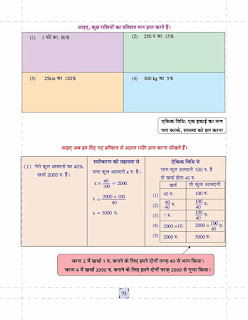Percentage worksheet in HindiPercentage worksheet in Hindi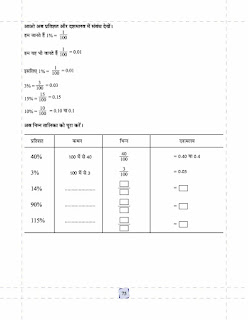decimal worksheet for Hindi medium.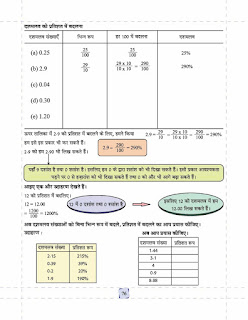decimal worksheet for Hindi medium.Profit & Loss worksheet for Hindi medium.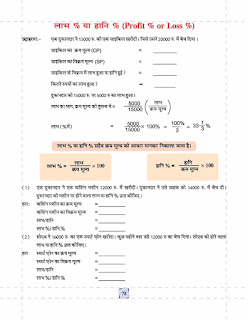Profit & Loss worksheet for Hindi medium.Download more worksheets.